# Image Similarity using Deep Ranking

The ability to find a similar set of images for a given image has multiple use-cases from visual search to duplicate product detection to domain specific image clustering. Especially in Google’s Reverse Image Search where the search engine retrieves similar images to your query image.

#### What is image similarity?

Image similarity is the measure of how similar two images are. In other words, it quantifies the degree of similarity between intensity patterns in two images.

#### How to build an image similarity model?

Initially, image similarity models considered category-level image similarity. For example, two images are considered similar as long as they belong to the same category. This category-level image similarity is not sufficient for the search-by-example image search application. Search by query image requires the distinction of differences between images within the same category- to recognise the fine grained differences in the similar images.

One way of buiding image similarity model is to extract features such as Gabor filters, SIFT , Local Binary Patterns, HOG  and then use these feature to compute the similarity between the features. The other way is to use deep learning model to aid in identifying similar images for a query image. Today, we will talk about a model called Deep Ranking , which learns fine-grained image similarity by characterising the fine-grained image similarity relationship with a set of triplets.

#### What is a triplet?

A triplet contains a query image, a positive image, and a negative image, where the positive image is more similar to the query image than the negative image. The below image illustrates this:

In the above image, each column is a triplet. The upper, middle and lower rows correspond to query image, positive image, and negative image- where the positive image is more similar to the query image that the negative image.

The image similarity relationship is characterized by relative similarity ordering in the triplets. Deep ranking models can employ this fine-grained image similarity information, which is not considered in category-level image similarity models or classification models, to achieve better performance.

#### How is image similarity different from image classification?

Let’s say you are looking at three cars parked in a road- a black car, a white car and a dark-gray car. For image classification models, these three cars are all just cars. It doesn’t concern itself with the colour and other aspect of the car. For a similar image ranking model, it would look at the colours and other aspects of the cars as well. If a query image is a “black car”, the similar image ranking model would rank the “dark gray car” higher than the “white car”. Due to this, image classification models may not fit directly to task of distinguishing fine-grained image similarity. The authors of the Deep Ranking paper propose to learn fine-grained image similarity with a deep ranking model, which characterizes the fine-grained image similarity relationship with a set of triplets.

#### How to measure the similarity of two images?

The metric used to measure the similarity between images is probably the most important thing in building image similarity models. While there are different metrics one can use to define the similarity, the most popular ones are L1-norm (also known as Manhattan distance) and L2 norm( also known as Euclidean distance). This paper gives a really good explanation and a comparison between Manhattan and Euclidean distance in the context of image similarity. Their results suggest that, in the domain of natural images of the kind we have used, the Manhattan distance may better capture the human notions of image similarity. In the case of Deep Ranking, the authors have used Squared Euclidean distance as the similarity metric. The smaller the distance the more similar the images are.

Note: Unfortunately, Medium editor doesn’t support super-scripting and sub-scripting for letters. ‘Pi+’ means ‘P’ subscript ‘i’ with superscript ‘+’ and ‘Pi-’ means ‘P’ subscript ‘i’ with superscript ‘-’.

#### The loss function

Neural Networks are universal function approximators. The whole Deep Ranking architecture can be thought of as a function that would map the image to a point in the Euclidean space. The goal is to learn an embedding function that assigns smaller distance to more similar images. This can be expressed as :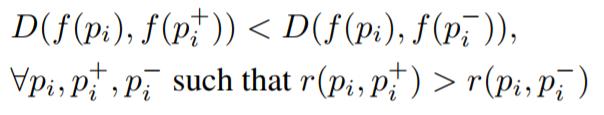Mathematical representation of the goal of the embedding function.

Here, ‘f’ is the embedding function to would map the image to a vector. Pi is the query image, Pi+ is the positive image, Pi- is the negative image and ‘r’ is the similarity distance between two images.

The hinge loss for the triplet is defined as:

Where ‘l’ is the hinge loss for the triplet, ‘g’ is a gap parameter that regularizes the gap between the distance of the two image pairs: ( Pi , Pi+ ) and ( Pi , Pi- ), and ‘D’ is the euclidean distance between the two euclidean points.

Here, you are optimising the model in such a way that the distance between query image and positive image is not only lesser than the distance between query image and negative, but is lesser by an amount of ‘g’.

#### Network Architecture

The most crucial component is to learn an image embedding function ‘f’. Traditional methods typically employ hand-crafted visual features, and learn linear or nonlinear transformations to obtain the image embedding function. Here, a deep learning technique is employed to learn image similarity models directly from images. The Deep Ranking network looks like this:

The network consists of 3 parts- The triplet sampling, the ConvNet and the Image Similarity ranking.

This network takes image triplets as input. One image triplet contains a query image ‘pi’ , a positive image ‘pi+’ and a negative image ‘pi-’ , which are fed independently into three identical deep neural networks ‘f(.)’ with shared architecture and parameters. A triplet characterizes the relative similarity relationship for the three images. The deep neural network ‘f(.)’ computes the embedding of an image ‘pi’ : f(pi) ∈ Rd , where ‘d’ is the dimension of the feature embedding, and ‘R’ represents the Real number space.

The ranking layer on the top evaluates the hinge loss of a triplet. During learning, it evaluates the model’s violation of the ranking order, and back-propagates the gradients to the lower layers so that the lower layers can adjust their parameters to minimize the ranking loss.

The ConvNet in the image above is a novel multi-scale deep neural network architecture that employs different levels of in-variance at different scales.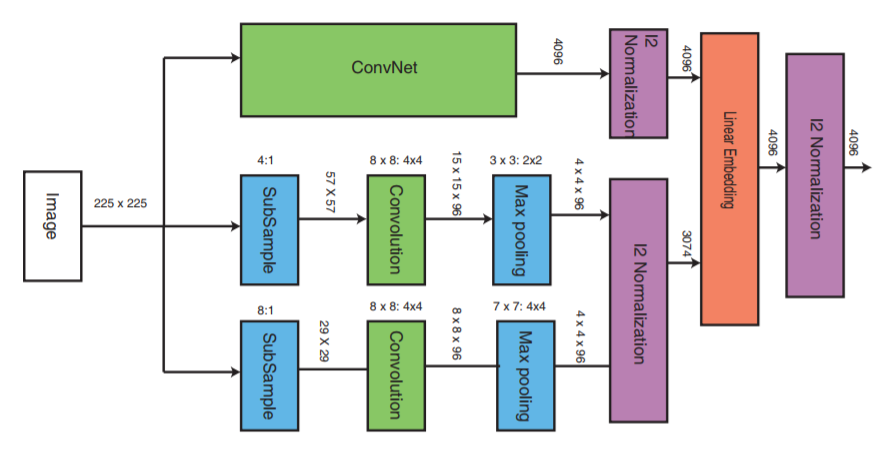Visual illustration of the ConvNet in the network architecture

The ConvNet encodes strong invariance and captures the image semantics. The other two parts of the network take down-sampled images and use shallow network architecture. Those two parts have less invariance and capture the visual appearance. Finally, we normalize the embeddings from the three parts, and combine them with a linear embedding layer.

The ConvNet can be any Deep Neural Network. The ConvNet contains stacked convolutional layers, max-pooling layer, local normalization layers and fully-connected layers.

A convolutional layer takes an image or the feature maps of another layer as input, convolves it with a set of ‘k’ learnable kernels, and puts through the activation function to generate ‘k’ feature maps. The convolutional layer can be considered as a set of local feature detectors.

A max pooling layer performs max pooling over a local neighborhood around a pixel. The max pooling layer makes the feature maps robust to small translations.

A local normalization layer normalizes the feature map around a local neighborhood to have unit norm and zero mean. It leads to feature maps that are robust to the differences in illumination and contrast.

The stacked convolutional layers, max-pooling layer and local normalization layers act as translational and contrast robust local feature detectors. A fully connected layer computes a non-linear transformation from the feature maps of these local feature detectors.

The authors used AlexNet for the ConvNet. The dimension of the embedding is 4096 in this case. To avoid overfitting, dropout with probability of 0.6 is applied to all the fully connected layers

Although ConvNet achieves very good performance for image classification, the strong in-variance encoded in its architecture can be harmful for fine-grained image similarity tasks. The experiments conducted by the authors showed that the multi-scale network architecture outperforms single scale ConvNet in fine-grained image similarity task.

#### Triplet Sampling

An effective strategy to select the most important triplets for rank learning is crucial. Uniformly sampling the triplets is sub-optimal, because we are more interested in the top-ranked results returned by the ranking model. The authors employ an online importance sampling scheme to sample triplets.

Note: ‘ri,j’ is ‘r’ subscript ‘i,j’, ‘ci’ is ‘c’ subscript ‘i’, ‘Tp’ is ‘T’ subscript ‘p’ and ‘zi’ is ‘z’ subscript ‘i’.

Suppose we have a set of images ‘P’, and their pairwise relevance scores ri,j = r(pi , pj ). Each image ‘pi’ belongs to a category, denoted by ‘ci’ .The total relevance score of an image ‘ri’ is defined as: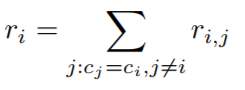Mathematical representation of the Total Relevance Score

The total relevance score of an image ‘pi’ reflects how relevant the image is in terms of its relevance to the other images in the same category.

Then, a positive image ‘pi+’ from the images sharing the same categories as ‘pi’ is sampled. Since the top-ranked images are more relevant and important, more positive images ‘pi+’ with high relevance scores ‘ri,i+’ are sampled. The probability of choosing an image ‘pi+’ as positive image is: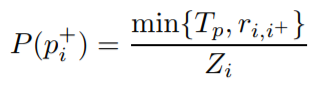Mathematical representation of the probability of positive image being chosen during sampling

where ‘Tp’ is a threshold parameter, and the normalization constant ‘Zi’ equals the sum of the probability of the positive image, sharing the the same categories with ‘pi’, being selected.

There two types of negative image samples. The first type is out-of-class negative samples, which are the negative samples that are in a different category from query image ‘pi’. They are drawn uniformly from all the images with different categories with ‘pi’. The second type is in-class negative samples, which are the negative samples that are in the same category as ‘pi’ but is less relevant to ‘pi’ than ‘pi+’. In order to ensure robust ordering between ‘pi+’ and ‘pi-’ in a triplet ti = (pi , pi+ , pi- ), it is also required that the margin between the relevance score ‘ri,i+’ and ‘ri,i-’ should be larger than ‘Tr’ , that is: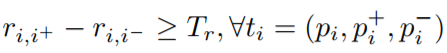Mathematical representation of the margin between the relevance score

Note: ‘kj’ is ‘k’ subscript ‘j’, ‘uj’ is ‘u’ subscript ‘j’, ‘pj/’ is ‘p’ subscript ‘j’ with superscript ‘/’. ‘/’ is just a dash.

Learning deep ranking models requires large amount of data, which cannot be loaded into main memory. The sampling algorithms that require random access to all the examples in the dataset cannot be used. The authors propose an online triplet sampling algorithm:

1. Create a set of buffers to store images. Each buffer has a fixed capacity, and it stores images from the same category.
2. For a new image ‘pj’, its key ‘kj’ = uj(1/rj),where ‘rj’ is its total relevance score and ‘uj’ is a uniformly sampled number from 0 to 1.
3. The buffer corresponding to the image ‘pj’ can be found according to its category ‘cj’ . If the buffer is not full, the image ‘pj’ is inserted into the buffer with key ‘kj’ . Otherwise, the image ‘pj/’ with smallest key ‘kj/’ in the buffer is selected.
4. If kj > kj/ , the image ‘pj/’ is replaced with image ‘pj’ in the buffer. Otherwise, the image ‘pj’ is discarded. If this replacing scheme is employed, uniformly sampling from a buffer is equivalent to drawing samples with probability proportional to the total relevance score ‘rj’.
5. One image ‘pi’ is uniformly sampled from all the images in the buffer of category ‘cj’ as the query image. Then one image, ‘pi+’, is uniformly generated from all the images in the buffer of category ‘cj’. This image acts as the positive image.
6. For a negative image sample, ‘pi-’ is drawn uniformly from all the images in the other buffers in the case of out-of-class negative image sample. For in-class negative image samples, the same as point 5 is used. But, it is accepted only if it satisfies the margin constraint ‘Tr’.

Whether in-class or out-of-class negative samples are sampled is controlled by a out-of-class sample ratio parameter. An illustration of this sampling method is shown below: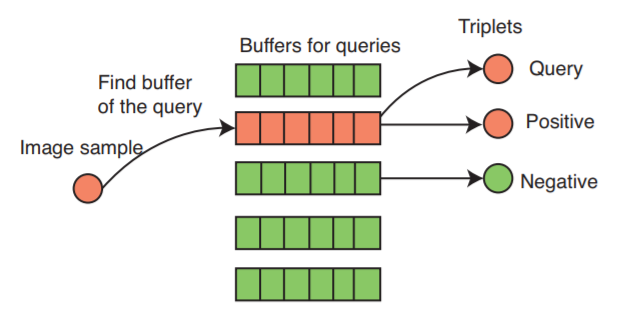Visual representation of how the images are sampled from the buffer

#### Training Data

The authors used two sets of training data to train the model. The first training data was ImageNet ILSVRC-2012 dataset, which contains roughly 1000 images in each of 1000 categories. In total, there are about 1.2 million training images, and 50,000 validation images. This dataset is utilized to learn image semantic information. They used it to pre-train the “ConvNet” part of the model using soft-max cost function as the top layer.

The second training data is the relevance training data, responsible for learning fine-grained visual similarity. It was collected from 100,000 search queries (using Google image search), with the top 140 image results from each query. There were about 14 million images. The relevance score for the images was computed using a golden feature. The golden feature is a weighted linear combination of twenty seven features. For the details of the golden features, please refer Section 6.1 of the original paper.

#### Comparison of Deep Ranking with Hand-crafted Features

The deep ranking model is compared with hand-crafted features. For each hand-crafted feature, its performance using its best experimental setting is shown below. The evaluated hand crafted visual features include Wavelet, Color (LAB histogram), SIFT-like features, SIFT-like Fisher vectors, HOG, and SPMK Taxton features with max pooling . Along with these, two image similarity models- L1HashKCPA and OASIS- are learned on top of the concatenation of all these visual features. The performance comparison is shown below.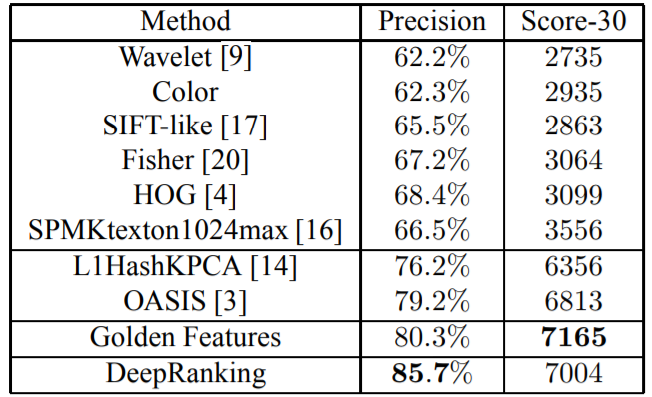Performance comparison of different features against Deep Ranking

We can see that any individual feature without learning does not perform very well. The L1HashKCPA feature achieves reasonably good performance with relatively low dimension, but its performance is inferior to Deep Ranking model. The OASIS algorithm can learn better features because it exploits the image similarity ranking information in the relevance training data. By directly learning a ranking model on images, the deep ranking method can use more information from image than two-step- feature extraction and model learning approach. Thus, it performs better both in terms of similarity precision and score-at-top-30.

The Deep Ranking model performs better in terms of similarity precision than the golden features, which are used to generate relevance training data. This is because the Deep Ranking model employs the category-level information in ImageNet data and relevance training data to better characterize the image semantics. The score-at-top-30 metric of Deep Ranking is only slightly lower than the golden features.

#### Comparison of Deep Ranking with Different Architectures

The Deep Ranking model is compared with the following architectures:

1. Deep neural network for classification trained on ImageNet, called ConvNet. This is exactly the same as the model trained in AlexNet.
2. Single-scale deep neural network for ranking. It only has a single scale ConvNet in deep ranking model, but it is trained in the same way as Deep Ranking model.
3. Train an OASIS model on the feature output of single-scale deep neural network for ranking.
4. Train a linear embedding on both the single scale deep neural network and the visual features described in the last section.

The performances are shown below: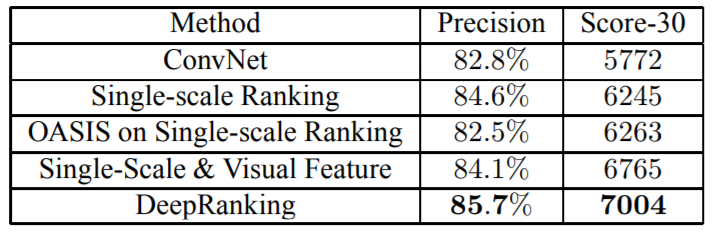Performance comparison of various architectures against Deep Ranking

In all the experiments, the Euclidean distance of the embedding vectors of the penultimate layer before the final softmax or ranking layer is exploited as similarity measure.

The ranking model greatly increases the performance. The performance of single-scale ranking model is much better than ConvNet. The two networks have the same architecture except the single-scale ranking model is fine-tuned with the relevance training data using ranking layer, while ConvNet is trained solely for classification task using logistic regression layer.

The single-scale ranking performs very well in terms of similarity precision, but its score-at-top-30 is not very high. The Deep Ranking model, which employs multi-scale network architecture, has better similarity precision and score-at-top-30. Finally, although training an OASIS model or linear embedding on the top increases performance, their performance is inferior to Deep Ranking model, which uses back-propagation to fine-tune the whole network.

#### Comparison of Different Sampling Methods

One very important experiments the authors ran were to see how the fraction of the out-of-class negative samples in online triplet sampling algorithm affected the performance. The score-at-top-30 metric of Deep Ranking model decreases as more out-of-class negative samples. However, having a small fraction of out-of-class samples (like 20%) increases the similarity precision metric a lot. You can see how the performance decreases as the fraction of out-of-class samples are increased in the below graph: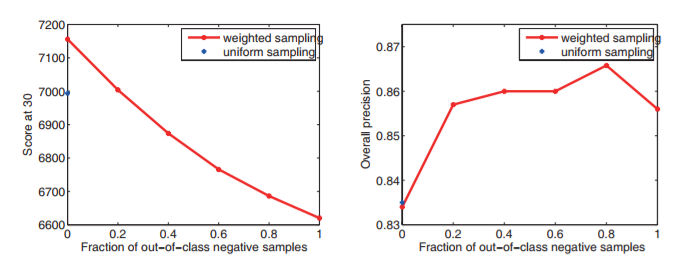Graphical representation of Score-at-30/Precision w.r.t Fraction of out-out-sample negative samples

They also compared the performance of the weighted sampling and uniform sampling with 0% out-of-class negative samples. In weighted sampling, the sampling probability of the images is proportional to its total relevance score ‘rj’ and pairwise relevance score ‘ri,j’, while uniform sampling draws the images uniformly from all the images (but the ranking order and margin constraints should be satisfied). Although the two sampling methods perform similarly in overall precision, the weighted sampling algorithm does better in score-at-top-30.

#### Ranking Examples

A comparison of the ranking examples of ConvNet, OASIS feature (L1HashKPCA features with OASIS learning) and Deep Ranking is shown below: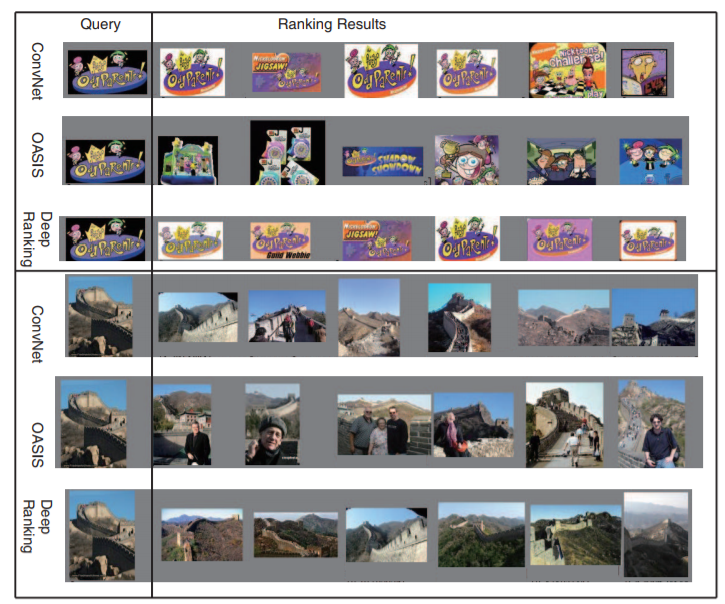Image Similarity ranking examples of different architectures

The ConvNet captures the semantic meaning of the images very well, but it fails to take into account some global visual appearance, such as color and contrast. On the other hand, Oasis features can characterize the visual appearance well, but fall short on the semantics. The proposed deep ranking method incorporates both the visual appearance and image semantics.

#### Where to find the dataset used in the paper?

The dataset is available here. Unfortunately, only 5,033 triplets are available as opposed to the 14,000 triplets used in the paper. The reason they aren’t available is because the public URLs for those images are no longer valid.

#### Implementation

I have implemented this model using Keras library. The tricky part with the implementation is the 3 parallel networks for query, positive and negative image. This is the one I am talking about. There are 3 major parts in the implementation:

1. Three parallel multi-scale network. Remember each network will again have 3 parallel networks- ConvNet and the 2 small networks. Having these three parallel multi-scale networks will be very expensive on the memory front. Especially when you are running this on the GPU.
2. We would have to write our own loss function. This loss function would take the output embedding of the 3 parallel multi-scale network and compute the hinge loss.
3. Generation of the triplets.

Unfortunately, the relevance score for the images are not available. So, we are going use a slightly modified version of the sampling method described in the paper. We will use the following method:

1. Each image in the given class forms the query image.
2. Each image other than the query image will form the positive image. But we can limit the number of positive images for each query image.
3. The negative image will be randomly chosen from any of the classes that is not the query image’s class.

The implementation is modular enough to change this algorithm. What we will do is, instead of sampling the triplets on the go, we will output the triplet into a text file where each line will contain the triplet in the order of query image, positive image and the negative image. Since the main program looks at this text file for the triplets, you can easily use other sampling techniques to generate this text file.

Training 3 parallel multi-scale network would be very memory expensive. If we can somehow train only 1 multi-scale network that would be equivalent to training 3 parallel multi-scale network would reduce the memory cost by a factor of 3. What we will do is, instead of having 3 parallel multi-scale network which takes query image, positive image and negative image at the same time, we will pass the query image, positive image and negative image sequentially to 1 multi-scale network. We know that the loss function sees batch size number of images to compute loss, so we use the loss function to identify with embedding belong to query image, positive image and negative image and calculate the hinge loss. The tensor passed onto the loss layer will contain the image embedding in each row. Each row corresponds to each input image in the batch. Since, we passed the query image, positive image and negative image sequentially, the first row will correspond to query image, the second to positive image and the third to negative images, and then same repeats until the end of the batch. This way, in the loss function, we get the embedding of all images and we just have to compute the hinge loss.

To implement the multi-scale network where the outputs of the ConvNet and the 2 small networks we will have to use the Merge layer in Keras. We will use the concatenate layer to concatenate the outputs of the ConvNet and the 2 small network. The code snippet for merging:

`merge_one = concatenate([first_max, second_max]) # To merge the two small networks 'first' and 'second'.`
`merge_two = concatenate([merge_one, convnet_model.output]) # To merge the ConvNet with the 2 small networks.`

To implement the loss function, we will have to write a custom loss function that would compute the euclidean distance between the query image and the positive image, and the euclidean distance between the query image and the negative image. And then would calculate the hinge loss. The snippet of the loss function:

`_EPSILON = K.epsilon()def _loss_tensor(y_true, y_pred):    y_pred = K.clip(y_pred, _EPSILON, 1.0-_EPSILON)    loss =  tf.convert_to_tensor(0,dtype=tf.float32) # initialise the loss variable to zero    g = tf.constant(1.0, shape=, dtype=tf.float32) # set the value for constant 'g'    for i in range(0,batch_size,3):        try:            q_embedding = y_pred[i+0] # procure the embedding for query image            p_embedding =  y_pred[i+1] # procure the embedding for positive image            n_embedding = y_pred[i+2] # procure the embedding for negative image            D_q_p =  K.sqrt(K.sum((q_embedding - p_embedding)**2)) # calculate the euclidean distance between query image and positive image            D_q_n = K.sqrt(K.sum((q_embedding - n_embedding)**2)) # calculate the euclidean distance between query image and negative image            loss = (loss + g + D_q_p - D_q_n ) # accumulate the loss for each triplet                   except:            continue    loss = loss/(batch_size/3) # Average out the loss     zero = tf.constant(0.0, shape=, dtype=tf.float32)    return tf.maximum(loss,zero)`

NOTE: The batch size should always be a multiple of 3. Since the triplet contains 3 images and the triplet images are passed sequentially, the batch size has to be multiple of 3.

To pass the path of the triplet text file to the Image Generator, the Image Generator class has been modified to take the path of the triplet text file as an input. So, to the Image Generator’s flow_from_directory, you will pass the path of the triplet text file to ‘triplet_file’ variable.

Here’s the snippet:

`class DataGenerator(object):    def __init__(self, params, target_size=(224, 224)):        self.params = params        self.target_size = target_size        self.idg = ImageDataGeneratorCustom(**params)    def get_train_generator(self, batch_size):        return self.idg.flow_from_directory("./dataset/",                                            batch_size=batch_size,                                            target_size=self.target_size,shuffle=False,                                            triplet_path  ='./triplet_5033.txt'                                           )    def get_test_generator(self, batch_size):        return self.idg.flow_from_directory("./dataset/",                                            batch_size=batch_size,                                            target_size=self.target_size, shuffle=False,                                            triplet_path  ='./triplet_5033.txt'                                        )`

Note: The shuffle parameter should be set to False.

That’s it! We are done with the implementation! You can find the whole code here.

Credits

All the credits go to the authors of the Deep Ranking (Learning Fine-grained Image Similarity with Deep Ranking) paper. I have merely explained their work and implemented it. This post wouldn’t have been possible without them :)

#### References

 Object Recognition from Local Scale-Invariant Features- http://www.cs.ubc.ca/~lowe/papers/iccv99.pdf

 Histograms of Oriented Gradients for Human Detection- https://courses.engr.illinois.edu/ece420/fa2017/hog_for_human_detection.pdf

 Learning Fine-grained Image Similarity with Deep Ranking- https://static.googleusercontent.com/media/research.google.com/en//pubs/archive/42945.pdf

 ImageNet Classification with Deep Convolutional Neural Networks- https://papers.nips.cc/paper/4824-imagenet-classification-with-deep-convolutional-neural-networks.pdf

 Dropout: A Simple Way to Prevent Neural Networks from Overfitting- https://www.cs.toronto.edu/~hinton/absps/JMLRdropout.pdf

 ImageNet: A Large-Scale Hierarchical Image Database- http://www.image-net.org/papers/imagenet_cvpr09.pdf

 Fast Multiresolution Image Querying- https://grail.cs.washington.edu/projects/query/mrquery.pdf

 Large-Scale Image Retrieval with Compressed Fisher Vectors- http://citeseerx.ist.psu.edu/viewdoc/download?doi=10.1.1.401.9140&rep=rep1&type=pdf

 Beyond Bags of Features: Spatial Pyramid Matching for Recognizing Natural Scene Categories- http://ieeexplore.ieee.org/document/1641019/

 Improved Consistent Sampling, Weighted Minhash and L1 Sketching- https://static.googleusercontent.com/media/research.google.com/en//pubs/archive/36928.pdf

 Large scale online learning of image similarity through ranking- http://jmlr.csail.mit.edu/papers/volume11/chechik10a/chechik10a.pdf

 Learning Fine-grained Image Similarity with Deep Ranking- https://users.eecs.northwestern.edu/~jwa368/pdfs/deep_ranking.pdf